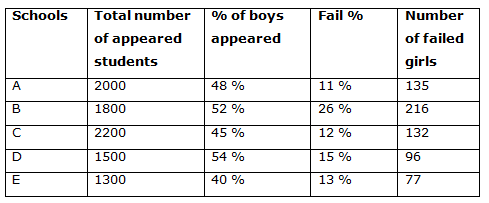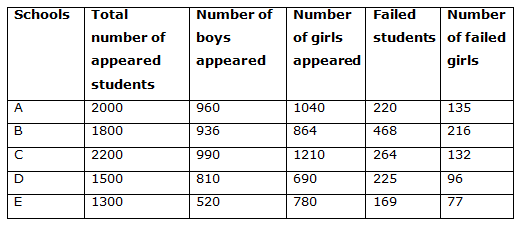# IBPS PO Mains 2018 – Quantitative Aptitude Questions Day-27

Dear Readers, Bank Exam Race for the Year 2018 is already started, To enrich your preparation here we have providing new series of Practice Questions on Quantitative Aptitude – Section. Candidates those who are preparing for IBPS PO Mains 2018 Exams can practice these questions daily and make your preparation effective.

[WpProQuiz 4291]

1) Two Stations A and B are 462 km apart. A train leaves station A from station B and at the same time another train leaves station B to A. Both the trains meet 5.5 hours after they start moving. If the train starts from station A is 28 km/hr faster than the other one, then find the ratio of the speed of train A to that of train B?

a) 2: 1

b) 3: 4

c) 5: 3

d) 11: 7

e) None of these

2) Rajesh can do a work in 36 days. He works alone for 8 days and Yuva alone finishes the remaining work in 21 days. Both of them together can complete the work in?

a) 12 5/9 days

b) 13 2/5 days

c) 14 4/11 days

d) 15 3/7 days

e) None of these

3) Raju, Vinitha and Agalya enter into a Partnership with investment in the ratio of (5/2): (9/5): (4/3). After six months Raju increases his share by 40 %. If the total profit at the end of the year be Rs. 47104, then what will be the share of Vinitha in the profit?

a) Rs. 15278

b) Rs. 13824

c) Rs. 11526

d) Rs. 14312

e) None of these

4) Train A can cross a pole in 2 sec. It can cross another train B of equal length coming from opposite direction with the speed of 42 m/s in 3 sec. Find the length (in m) and speed of the train A (in m/s)?

a) 234 m, 108 m/s

b) 216 m, 114 m/s

c) 252 m, 126 m/s

d) 308 m, 92 m/s

e) None of these

5) Pipe P and Q fill the tank in 15 mins and 30 mins respectively. If both the pipes are opened, after how much time Q should be closed so as to fill the tank in 11 min?

a) 6 min

b) 5 min

c) 8 min

d) 9 min

e) None of these

Directions (Q. 6 – 10) Study the following information carefully and answer the given questions:

The following table shows the number of students appeared for the certain examination from different schools.6) Find the ratio between the total number of failed boys in school A and B together to that of total failed students in school D and E together?

a) 152: 93

b) 223: 155

c) 344: 197

d) 23: 11

e) None of these

7) Find the difference between the total appeared girls from School B and D together to that of total appeared boys from School A and E together?

a) 74

b) 82

c) 96

d) 65

e) None of these

8) Find the average number of failed boys in all the given schools together?

a) 112

b) 126

c) 144

d) 138

e) None of these

9) In which school, the difference between the appeared boys and girls is maximum?

a) School C

b) School E

c) School A

d) School B

e) School D

10) Find the total number of passed girls in all the given schools together?

a) 4123

b) 2956

c) 3928

d) 3327

e) None of these

Let the speed of the train A be x and the speed of train B be y,

Then, x – y = 28 –à(i)

Time = Distance/Speed

5.5 = 462/(x + y)

x + y = 462/5.5

x + y = 84 –à(ii)

By solving (i) and (ii), we get,

2x = 112

X = 56, y = 28

The ratio of their speed = 56: 28 = 2: 1

Rajesh’s one day work = (1/36)

Rajesh’s 8 day work = (8/36) = 2/9

Remaining work = 1- 2/9 = 7/9

(7/9)*Yuva’s whole work = 21

Yuva’s whole work = 21*(9/7) = 27

Yuva’s one day work = 1/27

(Rajesh + Yuva)’s one day work = (1/36) + (1/27)

= > (27 + 36)/(36*27)

= > 7/108

(Rajesh + Yuva)’s whole work = 108/7 = 15 3/7 days

The investment ratio = (5/2): (9/5): (4/3)

= > 75: 54: 40

The share of Raju, Vinitha and Agalya

= > [75*6 + 75*(140/100)*6] : [54*12] : [40*12]

= > 1080: 648: 480

= > 90: 54: 40

Total profit = Rs. 47104

184’s = 47104

1’s = 256

The share of Vinitha = 54*256 = Rs. 13824

Let the length of the train A be l m,

And the speed of the train A = s m/s

According to the question

l = 2s —à (i)

l + l = (s + 42)*3

= > 2l = (s + 42)*3

= > 2*2s = 3s + 126

= > 4s – 3s = 126

= > s = 126 m/s

l = 2s = 126*2 = 252 m

Pipe P and Q’s one min work = (1/15) + (1/30)

After x min pipe Q closed, so,

11/15 + x/30 =1

22 + x = 30

X= 8

After 8 min, Pipe Q is closed.

Direction (6-10) :

The total number of failed boys in school A and B together

= > 2000*(11/100) + 1800*(26/100)

= > 220 + 468 = 688

The total failed students in school D and E together

= > 1500*(15/100) + 1300*(13/100)

= > 225 + 169 = 394

Required ratio = 688: 394 = 344: 197

The total appeared girls from School B and D together

= > 1800*(48/100) + 1500*(46/100)

= > 864 + 690 = 1554

The total appeared boys from School A and E together

= > 2000*(48/100) + 1300*(40/100)

= > 960 + 520 = 1480

Required difference = 1554 – 1480 = 74The total number of failed boys in all the given schools together

= > (220 – 135) + (468 – 216) + (264 – 132) + (225 – 96) + (169 – 77)

= > 85 + 252 + 132 + 129 + 92 = 690

Required average = 690/5 = 138In School A, Required difference = 1040 – 960 = 80

In School B, Required difference = 936 – 864 = 72

In School C, Required difference = 1210 – 990 = 220

In School D, Required difference = 810 – 690 = 120

In School E, Required difference = 780 – 520 = 260The total number of passed girls in all the given schools together

= > (1040 – 135) + (864 – 216) + (1210 – 132) + (690 – 96) + (780 – 77)

= > 905 + 648 + 1078 + 594 + 703 = 3928

Daily Practice Test Schedule | Good Luck

 Topic Daily Publishing Time Daily News Papers & Editorials 8.00 AM Current Affairs Quiz 9.00 AM Current Affairs Quiz (Hindi) 9.30 AM IBPS Clerk Prelims – Reasoning 10.00 AM IBPS Clerk Prelims – Reasoning (Hindi) 10.30 AM IBPS Clerk Prelims – Quantitative Aptitude 11.00 AM IBPS Clerk Prelims – Quantitative Aptitude (Hindi) 11.30 AM Vocabulary (Based on The Hindu) 12.00 PM IBPS Clerk Prelims – English Language 1.00 PM SSC Practice Questions (Reasoning/Quantitative aptitude) 2.00 PM IBPS PO/Clerk – GK Questions 3.00 PM SSC Practice Questions (English/General Knowledge) 4.00 PM Daily Current Affairs Updates 5.00 PM IBPS PO Mains – Reasoning 6.00 PM IBPS PO Mains – Quantitative Aptitude 7.00 PM IBPS PO Mains – English Language 8.00 PM